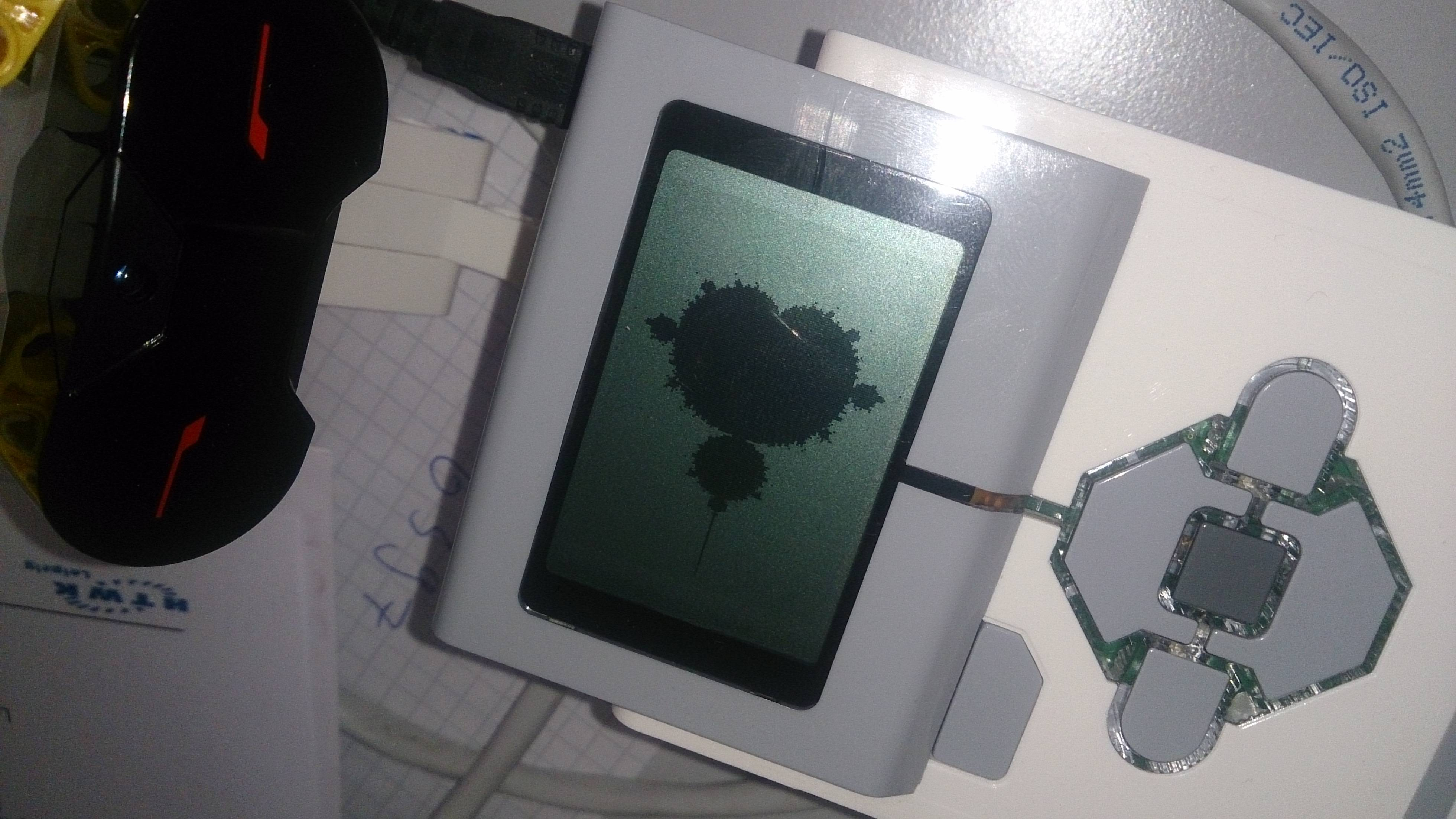Published: Mon 05 December 2016

Clojure and the Mandelbrot set on LEGO Mindstorms EV3

The LeJOS operating system for LEGO Mindstorms EV3 is, as I said before, a small Linux with an embedded JVM.

Running Clojure:

To run Clojure on the EV3 I had to download the Clojure runtime, unzip it and throw away everything but the actual jar-file clojure-1.8.0.jar.

Then the jar-file needs to be transfered to the EV3 (via scp for example) and can be executed via ssh with jrun -jar clojure-1.8.0.jar (I already have an alias in my ~/.ssh/config for the EV3). After about two minutes we have a Clojure repl that we can use to answer the important questions of our time.Takes about two minutes to start the repl

Actually coding something:

The first thing we have to notice is, that the leJOS menu stays visible when we opened the clojure repl. This is a problem insofar, that when we use the Clojure code to interact with (for example) the display, both the leJOS menu and our application want to use the display and their interactions will overlap in unpredictable ways (for me both images were "fighting", but the system might as well crash).

So I created a shell script that kills the existing java process and opens it again once our command ran.

#!/bin/sh
killall java
jrun # ... we will look at that later

Now we want to actually write Clojure code where we calculate members of the Mandelbrot set. The Mandelbrot set is the set of all numbers $$c$$ for which $$z_n$$ with $$z_0 = 0$$ and $$z_{n+1} = z_{n}^2 + c$$ does not diverge (when $$n$$ goes to infinity). This is calculated in the complex plane. The numbers for which this doesn't diverge are usually drawn in black while the diverging numbers remain white.

I looked for a Java-based library for the complex numbers and found one with Apache. This library was insofar underwhelming, that taking a complex number to the power of an arbitrary Integer doesn't work as one expects. The library always uses the following equivalence: $$y^x = exp(x \times log(y))$$ which is fine in general but doesn't work if $$y$$ is zero, which is the base case but also no problem with positive integer powers. Took me an hour because that is not at all well documented (for the integer-case). So the first thing is, to write our own pow function: (defn pow [y, x] (if (.equals y Complex/ZERO) y (.pow y x)))

Now we can define both $$z_n$$ and whether any $$z_n$$ diverges:

(defn zn [n, c] ( if (== n 0) Complex/ZERO (.add c (pow (zn (- n 1) c) 2.0 ) ) ))
(defn diverges [x] (or (.isNaN x) (.isInfinite x) (< 2.0 (.abs x))))

And the idea is, to set the whole display to black and evaluate for every black pixel $$z_1$$ and then for every remaining black pixel $$z_2$$. Once a pixel was set to white, we no longer need to evaluate it, because we already know it diverges.

So here's the full code for mb.clj:

(import [org.apache.commons.math3.complex Complex])
(import [lejos.hardware.lcd LCD])
(import [lejos.utility Delay])

(defn pow [y, x] (if (.equals y Complex/ZERO) y (.pow y x) ))
(defn zn [n, c] ( if (== n 0) Complex/ZERO (.add c (pow (zn (- n 1) c) 2.0 ) ) ))
(defn diverges [x] (or (.isNaN x) (.isInfinite x) (< 2.0 (.abs x))))

(defn scale_x [x] ( - (/ (* 2.5 x) LCD/SCREEN_WIDTH ) 2.0 ))
(defn scale_y [y] ( - (/ (* 2.0 y) LCD/SCREEN_HEIGHT) 1.0 ))

(doseq [x (range  LCD/SCREEN_WIDTH )
y (range  LCD/SCREEN_HEIGHT)] (LCD/setPixel x y 1))

(doseq [rep (range 1 1000)]
(doseq [x (range  LCD/SCREEN_WIDTH )
y (range  LCD/SCREEN_HEIGHT)]
(
if (== 1 (LCD/getPixel x y))
(if (diverges (zn rep (Complex. (scale_x x) (scale_y y)))) (LCD/setPixel x y 0))
)
)
)

We don't need a delay at the end because this will take long enough.

But now we have to think about running this. Basically we need to include every jar we use into the java classpath and then run the clojure main with our script as the parameter. For org.apache.commons.math3 we need the commons-math3-3.6.1.jar from Apache and for the lejos namespace we need the ev3classes.jar from leJOS (which is not included on the system because it is usually compiled into the finished jar).

Once this is all done we can basically run our application with jrun -cp "../ev3classes.jar:./commons-math3-3.6.1.jar:../clojure-1.8.0.jar" clojure.main mb.clj.After a few hours, it will look like this

I am pretty sure it is possible to compile the whole jar with the correct main using eclipse/ant and whatnot. But that's the first successful experiment in this direction. Here's a timelapse of the program in action.

Comments and Discussion is provided by Disqus. They are tracking site and user interaction. Please refer to their privacy policy for information about data usage and retention. If you still want to look at comments or comment yourself, enable disqus by clicking here.

Previous: Upgrading Fedora 24 to 25 , Next: Mandelbrot set on EV3 Timelapse